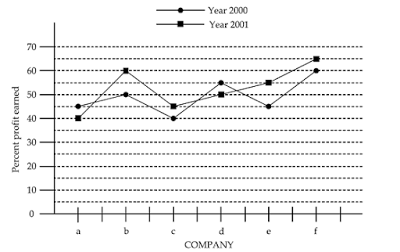UGC-NET/SET-PAPER-I(2006-DEC) - MODEL PAPER-49
ONLINE TEST NET/SET PAPER-1
Study the following graph and answer question numbers 31 to 33 :
2 points32. In the year 2001, which of the following Companies earned minimum percent profit ?
2 points
33. In the years 2000 and 2001, which of the following Companies earned maximum average percent profit ?
2 points
34. Human Development Report for ‘each’ of the year at global level has been published by:
2 points
35. The number of students in four classes A, B, C, D and their respective mean marks obtained by each of the class are given below : Class A Class B Class C Class DNumber of students 10 40 30 20Arithmetic mean 20 30 50 15The combined mean of the marks of four classes together will be :
2 points
36. LAN stands for :
2 points
37. Which of the following statement is correct ?
2 points
38. Which of the following is the appropriate definition of a computer ?
2 points
39. Information and Communication Technology includes :
2 points
40. Which of the following is the appropriate format of URL of e-mail ?
2 points